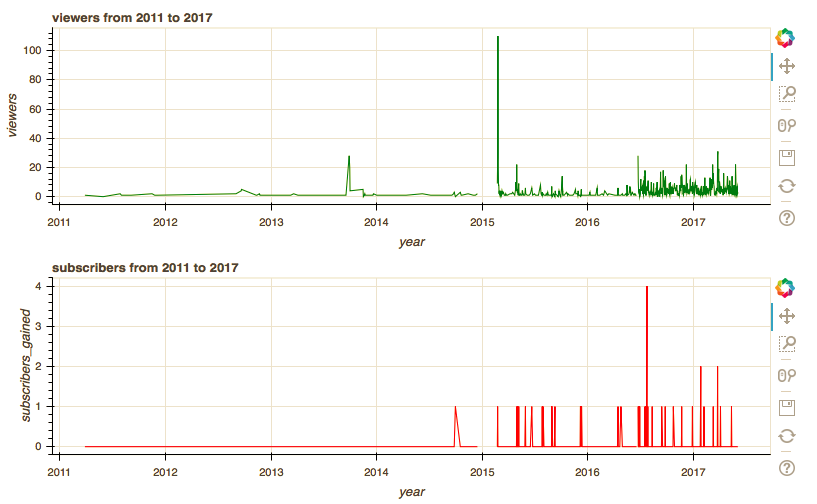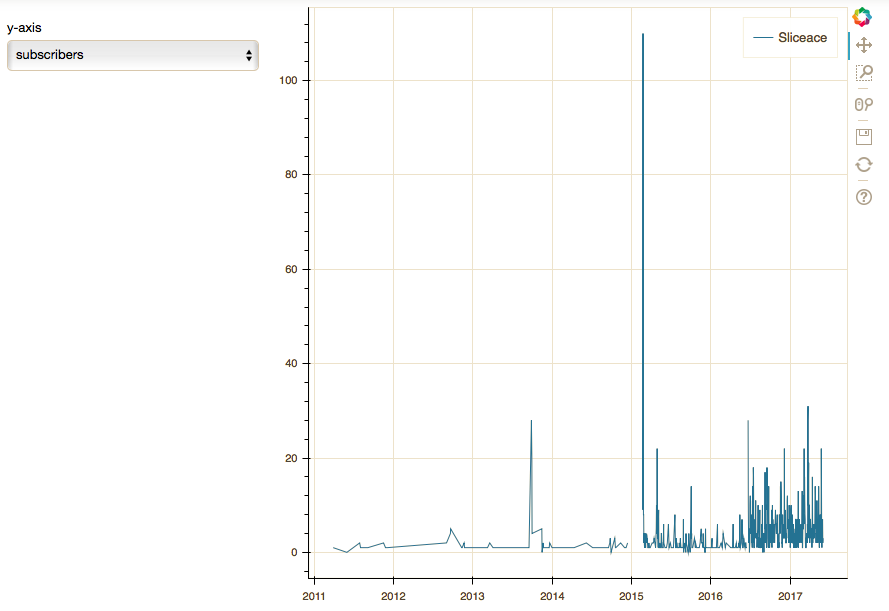# Changing y axis variables of the data set while keeping the x axis(date time object) constant

Hi,

I’ve been making a data visualisation tool for fun for my YouTube channel data. Initially, I tried to make a grid layout for the same x-axis and different y axis variables from the data frame i’ve attached ‘yt.csv’. On the other hand, i’m using the jupyter notebook to make these plots and Python 3.5, Pandas 0.20.2, Seaborn 0.7.1 and Bokeh 0.12.2. Here’s the code i used to make the plot i mention initially.

# Making the necessary imports

from bokeh.plotting import figure

from bokeh.io import output_file, output_notebook, show

from bokeh.layouts import column

from bokeh.models import HoverTool, ColumnDataSource

# convert column to a datetime

df1[‘date’] = pd.to_datetime(df1[‘date’])

df1[“date”]

# putting it in a fixed area

p = figure(plot_width = 800, plot_height = 250, title = “viewers from 2011 to 2017”,

x_axis_label = “year”, y_axis_label = “viewers”, x_axis_type =“datetime”)

p2 = figure(plot_width = 800, plot_height = 250,title = “subscribers from 2011 to 2017”,

x_axis_label = “year”, y_axis_label = “subscribers_gained”, x_axis_type =“datetime”)

p.line(df1[“date”], df1[“views”], color = “green”)

p2.line(df1[“date”], df1[“subscribers_gained”], color = “red”)

# put plots p1 and p2 on a column

layout = column(p,p2)

# embed plot to notebook

output_notebook()

# show result

show(layout)

This what i got.Then i thought what if i keep the x-axis constant and change the y-axis. Here’s an attempt. But notice the plot doesn’t update even after changing the variable of the y-axis. Here’s the code i used to generate it.

# Making the necessary imports

from bokeh.models import ColumnDataSource

from bokeh.models import Select

from bokeh.layouts import row

from bokeh.io import curdoc

from bokeh.layouts import widgetbox

from bokeh.models import Slider

# Create ColumnDataSource: source

source = ColumnDataSource(data={

‘x’ : df1[“date”],

‘y’ : df1[“views”]

})

# Create a plot

plot = figure(x_axis_type =“datetime”)

# Add lines to the plot

plot.line(‘x’, ‘y’, source = source, legend = “Sliceace”)

# Defining a callback function

def update_plot(attr, old, new):

# if the new selection is views

if new == “views”:

source.data = {

“x” : df1[“date”],

“y” : df1[“views”]

}

# else, update y

else:

source.data = {

“x” : df1[“date”],

“y” : df1[“subscribers”]

}

# remove the date column

columns = df1.drop(‘date’, axis = 1)

columns = sorted(df.columns)

# create a dropdown select widget

select = Select(title = “y-axis”, options = [‘likes’, ‘dislikes’, ‘subscribers_gained’, ‘subscribers’], value = “views”)

# Attach the update_plot callback to the ‘value’ property of select

select.on_change(“value”, update_plot)

# Create layout and add to current document

layout2 = row(select, plot)

output_notebook()

show(layout2)

The plot i gotNotice that the y-axis is the views column and is not updating. So my question is, how can i update the y-axis to draw different variables available in the dataset(“yt.csv”). While keeping the x-axis constant? And why isn’t my y-axis updating as i’m changing my Selection in the dropdown?

Regards,

Nyabuti Mainye.

yt.csv (92.5 KB)

I’ve decided to use `input()` function to try and answer my question. Otherwise, i’m still stuck using the dropdown select widget.# Making the necessary imports

from bokeh.plotting import figure
from bokeh.io import output_file, output_notebook, show
from bokeh.layouts import column
from bokeh.models import HoverTool, ColumnDataSource

# convert column to a datetime

df1[‘date’] = pd.to_datetime(df1[‘date’])

df1[“date”]

# Statement allowing user to label axes on their own.

print (“Label your graph for channel 1: start with the title e.g views for Sliceace channel from 2011 to 2017 and the y axis”)

# putting it in a fixed area

p = figure(plot_width = 800, plot_height = 250, title = input(“Please type in your title:”),
x_axis_label = “year”, y_axis_label = input(“Please type in your y axis label”), x_axis_type = “datetime”)

p2 = figure(plot_width = 800, plot_height = 250, title = “subscribers from 2011 to 2017”,
x_axis_label = “year”, y_axis_label = “subscribers_gained”, x_axis_type =“datetime”)

# Variables available to be plotted on the y axis

print(“Type exactly how you see it in the list above”)

p.line(df1[“date”], df1[input("")], color = “green”)
p2.line(df1[“date”], df1[“subscribers_gained”], color = “red”) # will add another channel here

# put plots p1 and p2 on a column

layout = column(p,p2)
output_notebook()
show(layout)

``

···

On Friday, August 4, 2017 at 8:32:03 AM UTC+3, Nyabuti M wrote:

Hi,

I’ve been making a data visualisation tool for fun for my YouTube channel data. Initially, I tried to make a grid layout for the same x-axis and different y axis variables from the data frame i’ve attached ‘yt.csv’. On the other hand, i’m using the jupyter notebook to make these plots and Python 3.5, Pandas 0.20.2, Seaborn 0.7.1 and Bokeh 0.12.2. Here’s the code i used to make the plot i mention initially.

# Making the necessary imports

from bokeh.plotting import figure
from bokeh.io import output_file, output_notebook, show
from bokeh.layouts import column
from bokeh.models import HoverTool, ColumnDataSource

# convert column to a datetime

df1[‘date’] = pd.to_datetime(df1[‘date’])

df1[“date”]

# putting it in a fixed area

p = figure(plot_width = 800, plot_height = 250, title = “viewers from 2011 to 2017”,
x_axis_label = “year”, y_axis_label = “viewers”, x_axis_type =“datetime”)

p2 = figure(plot_width = 800, plot_height = 250,title = “subscribers from 2011 to 2017”,
x_axis_label = “year”, y_axis_label = “subscribers_gained”, x_axis_type =“datetime”)

p.line(df1[“date”], df1[“views”], color = “green”)
p2.line(df1[“date”], df1[“subscribers_gained”], color = “red”)

# put plots p1 and p2 on a column

layout = column(p,p2)

# embed plot to notebook

output_notebook()

# show result

show(layout)

``

This what i got.Then i thought what if i keep the x-axis constant and change the y-axis. Here’s an attempt. But notice the plot doesn’t update even after changing the variable of the y-axis. Here’s the code i used to generate it.

# Making the necessary imports

from bokeh.models import ColumnDataSource
from bokeh.models import Select
from bokeh.layouts import row
from bokeh.io import curdoc
from bokeh.layouts import widgetbox
from bokeh.models import Slider

# Create ColumnDataSource: source

source = ColumnDataSource(data={
‘x’ : df1[“date”],
‘y’ : df1[“views”]
})

# Create a plot

plot = figure(x_axis_type =“datetime”)

# Add lines to the plot

plot.line(‘x’, ‘y’, source = source, legend = “Sliceace”)

# Defining a callback function

def update_plot(attr, old, new):
# if the new selection is views
if new == “views”:
source.data = {
“x” : df1[“date”],
“y” : df1[“views”]
}
# else, update y
else:
source.data = {
“x” : df1[“date”],
“y” : df1[“subscribers”]
}

# remove the date column

columns = df1.drop(‘date’, axis = 1)
columns = sorted(df.columns)

# create a dropdown select widget

select = Select(title = “y-axis”, options = [‘likes’, ‘dislikes’, ‘subscribers_gained’, ‘subscribers’], value = “views”)

# Attach the update_plot callback to the ‘value’ property of select

select.on_change(“value”, update_plot)

# Create layout and add to current document

layout2 = row(select, plot)

output_notebook()

show(layout2)

``

The plot i gotNotice that the y-axis is the views column and is not updating. So my question is, how can i update the y-axis to draw different variables available in the dataset(“yt.csv”). While keeping the x-axis constant? And why isn’t my y-axis updating as i’m changing my Selection in the dropdown?